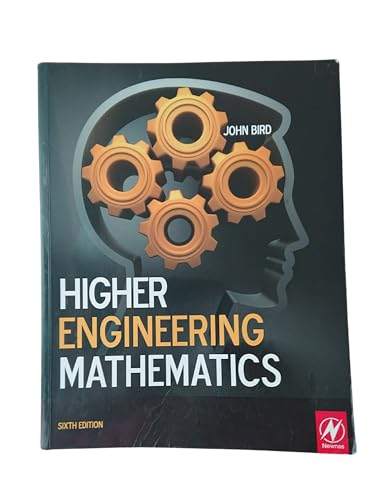# Higher Engineering Mathematics by John Bird

In Stock
\$72.99
+10% GST
Explains basic mathematical theories, supported by practical engineering examples and applications in order to ensure that readers can relate theory to practice. Introducing higher mathematical concepts from an engineering perspective, this title is mapped to BTEC Higher National Engineering and Foundation Degree unit specifications.
Only 1 left

## Higher Engineering Mathematics Summary

### Higher Engineering Mathematics by John Bird

Now in its sixth edition, Higher Engineering Mathematics is an established textbook that has helped many thousands of students to gain exam success. John Bird's approach is ideal for students from a wide range of academic backgrounds, and can be worked through at the student's own pace. Mathematical theories are examined in the simplest of terms, supported by practical examples and applications from a wide variety of engineering disciplines, to ensure that the reader can apply theory to practice. This extensive and thorough topic coverage makes this an ideal book for a range of university degree modules, foundation degrees, and HNC/D units.

This new edition of Higher Engineering Mathematics has been further extended with topics specifically written to help first year engineering degree students and those following foundation degrees. New material has been added on logarithms and exponential functions, binary, octal and hexadecimal numbers, vectors and methods of adding alternating waveforms.

This book caters specifically for the engineering mathematics units of the Higher National Engineering schemes from Edexcel, including the core unit Analytical methods for Engineers, and two optional units: Further Analytical Methods for Engineers and Engineering Mathematics, common to both the electrical/electronic engineering and mechanical engineering pathways. A mapping grid is included showing precisely which topics are required for the learning outcomes of each unit.

Higher Engineering Mathematics contains examples, supported by 900 worked problems and 1760 further problems contained within exercises throughout the text. In addition, 19 revision tests, which are available to use as tests or as homework are included at regular intervals.

### Why buy from World of BooksOur excellent value books literally don't cost the earthFree delivery in AustraliaEvery used book bought is one saved from landfill

John Bird, the author of over 100 textbooks on engineering and mathematical subjects, is the former Head of Applied Electronics in the Faculty of Technology at Highbury College, Portsmouth, U.K. More recently, he has combined freelance lecturing at Portsmouth University, with technical writing and Chief Examiner responsibilities for City and Guilds Telecommunication Principles and Mathematics, and examining for the International Baccalaureate Organisation. John Bird is currently a Senior Training Provider at the Royal Naval School of Marine Engineering in the Defence College of Marine and Air Engineering at H.M.S. Sultan, Gosport, Hampshire, U.K. The school, which serves the Royal Navy, is one of Europe's largest engineering training establishments.

Preface; Algebra; Partial fractions; Logarithms; Exponential functions; Hyperbolic functions; Arithmetic and geometric progressions; The binomial series; Maclaurin's series; Solving equations by iterative methods; Binary; octal and hexadecimal; Introduction to trigonometry; Cartesian and polar co-ordinates; The circle and its properties; Trigonometric waveforms; Trigonometric identities and equations; The relationship between trigonometric and hyperbolic functions; Compound angles; Functions and their curves; Irregular areas; volumes and mean values of waveforms; Complex numbers; De Moivre's theorem; The theory of matrices and determinants; The solution of simultaneous equations by matrices and determinants; Vectors; Methods of adding alternating waveforms; Scalar and vector products; Methods of differentiation; Some applications of differentiation; Differentiation of parametric equations; Differentiation of implicit functions; Logarithmic differentiation; Differentiation of hyperbolic functions; Differentiation of inverse trigonometric and hyperbolic functions; Partial differentiation; Total differential; rates of change and small changes; Maxima; minima and saddle points for functions of two variables; Standard integration; Some applications of integration; Integration using algebraic substitutions; Integration using trigonometric and hyperbolic substitutions; Integration using partial fractions; The t = __substitution; Integration by parts; Reduction formulae; Numerical integration; Solution of first order differential equations by separation of variables; Homogeneous first order differential equations; Linear first order differential equations; Numerical methods for first order differential equations; Second order differential equations of the form __; Second order differential equations of the form __; Power series methods of solving ordinary differential equations; An introduction to partial differential equations; Presentation of statistical data; Measures of central tendency and dispersion; Probability; The binomial and Poisson distributions; The normal distribution; Linear correlation; Linear regression; Introduction to Laplace transforms; Properties of Laplace transforms; Inverse Laplace transforms; The solution of differential equations using Laplace transforms; The solution of simultaneous differential equations using Laplace transforms; Fourier series for periodic functions of period 2p ; Fourier series for a non-periodic function over range 2p ; Even and odd functions and half-range Fourier series; Fourier series over any range; A numerical method of harmonic analysis; The complex or exponential form of a Fourier series; Essential formulae; Index

GOR005376279
Higher Engineering Mathematics by John Bird
John Bird
Used - Very Good
Paperback
Taylor & Francis Ltd
2010-04-12
704
185617767X
9781856177672
N/A
Book picture is for illustrative purposes only, actual binding, cover or edition may vary.
This is a used book - there is no escaping the fact it has been read by someone else and it will show signs of wear and previous use. Overall we expect it to be in very good condition, but if you are not entirely satisfied please get in touch with us.